Important: This notebook will only work with fastai-0.7.x. Do not try to run any fastai-1.x code from this path in the repository because it will load fastai-0.7.x

# Random Forest Model interpretation¶

In [ ]:
%load_ext autoreload

In [ ]:
%matplotlib inline

from fastai.imports import *
from fastai.structured import *
from pandas_summary import DataFrameSummary
from sklearn.ensemble import RandomForestRegressor, RandomForestClassifier
from IPython.display import display
from sklearn import metrics

In [ ]:
set_plot_sizes(12,14,16)


## Load in our data from last lesson¶

In [ ]:
PATH = "data/bulldozers/"

df_trn, y_trn, nas = proc_df(df_raw, 'SalePrice')

In [ ]:
def split_vals(a,n): return a[:n], a[n:]
n_valid = 12000
n_trn = len(df_trn)-n_valid
X_train, X_valid = split_vals(df_trn, n_trn)
y_train, y_valid = split_vals(y_trn, n_trn)
raw_train, raw_valid = split_vals(df_raw, n_trn)

In [ ]:
def rmse(x,y): return math.sqrt(((x-y)**2).mean())

def print_score(m):
res = [rmse(m.predict(X_train), y_train), rmse(m.predict(X_valid), y_valid),
m.score(X_train, y_train), m.score(X_valid, y_valid)]
if hasattr(m, 'oob_score_'): res.append(m.oob_score_)
print(res)

In [ ]:
df_raw

Out[ ]:
SalesID SalePrice MachineID ModelID datasource auctioneerID YearMade MachineHoursCurrentMeter UsageBand fiModelDesc ... saleDay saleDayofweek saleDayofyear saleis_month_end saleis_month_start saleis_quarter_end saleis_quarter_start saleis_year_end saleis_year_start saleElapsed
0 1139246 11.097410 999089 3157 121 3.0 2004 68.0 Low 521D ... 16 3 320 False False False False False False 6512
1 1139248 10.950807 117657 77 121 3.0 1996 4640.0 Low 950FII ... 26 4 86 False False False False False False 5547
2 1139249 9.210340 434808 7009 121 3.0 2001 2838.0 High 226 ... 26 3 57 False False False False False False 5518
3 1139251 10.558414 1026470 332 121 3.0 2001 3486.0 High PC120-6E ... 19 3 139 False False False False False False 8157
4 1139253 9.305651 1057373 17311 121 3.0 2007 722.0 Medium S175 ... 23 3 204 False False False False False False 7492
5 1139255 10.184900 1001274 4605 121 3.0 2004 508.0 Low 310G ... 18 3 353 False False False False False False 7275
6 1139256 9.952278 772701 1937 121 3.0 1993 11540.0 High 790ELC ... 26 3 239 False False False False False False 5700
7 1139261 10.203592 902002 3539 121 3.0 2001 4883.0 High 416D ... 17 3 321 False False False False False False 6148
8 1139272 9.975808 1036251 36003 121 3.0 2008 302.0 Low 430HAG ... 27 3 239 False False False False False False 7527
9 1139275 11.082143 1016474 3883 121 3.0 1000 20700.0 Medium 988B ... 9 3 221 False False False False False False 6778
10 1139278 10.085809 1024998 4605 121 3.0 2004 1414.0 Medium 310G ... 21 3 234 False False False False False False 7156
11 1139282 10.021271 319906 5255 121 3.0 1998 2764.0 Low D31E ... 24 3 236 False False False False False False 6428
12 1139283 10.491274 1052214 2232 121 3.0 1998 0.0 NaN PC200LC6 ... 20 3 293 False False False False False False 6120
13 1139284 10.325482 1068082 3542 121 3.0 2001 1921.0 Medium 420D ... 26 3 26 False False False False False False 6218
14 1139290 10.239960 1058450 5162 121 3.0 2004 320.0 Low 214E ... 3 1 3 False False False False False False 6195
15 1139291 9.852194 1004810 4604 121 3.0 1999 2450.0 Medium 310E ... 16 3 320 False False False False False False 6512
16 1139292 9.510445 1026973 9510 121 3.0 1999 1972.0 Low 334 ... 14 3 165 False False False False False False 6722
17 1139299 9.159047 1002713 21442 121 3.0 2003 0.0 NaN 45NX ... 28 3 28 False False False False False False 7681
18 1139301 9.433484 125790 7040 121 3.0 2001 994.0 Low 302.5 ... 9 3 68 False False False False False False 6260
19 1139304 9.350102 1011914 3177 121 3.0 1991 8005.0 Medium 580SUPER K ... 17 3 321 False False False False False False 6148
20 1139311 10.621327 1014135 8867 121 3.0 2000 3259.0 Medium JS260 ... 18 3 138 False False False False False False 6330
21 1139333 10.448715 999192 3350 121 3.0 1000 16328.0 Medium 120G ... 19 3 292 False False False False False False 6484
22 1139344 10.165852 1044500 7040 121 3.0 2005 109.0 Low 302.5 ... 25 3 298 False False False False False False 6855
23 1139346 11.198215 821452 85 121 3.0 1996 17033.0 High 966FII ... 19 3 292 False False False False False False 6484
24 1139348 10.404263 294562 3542 121 3.0 2001 1877.0 Medium 420D ... 20 3 141 False False False False False False 5602
25 1139351 9.433484 833838 7009 121 3.0 2003 1028.0 Medium 226 ... 9 3 68 False False False False False False 6260
26 1139354 9.648595 565440 7040 121 3.0 2003 356.0 Low 302.5 ... 9 3 68 False False False False False False 6260
27 1139356 10.878047 1004127 25458 121 3.0 2000 0.0 NaN EX550STD ... 22 3 53 False False False False False False 6610
28 1139357 10.736397 44800 19167 121 3.0 2004 904.0 Low 685B ... 9 3 221 False False False False False False 6778
29 1139358 11.396392 1018076 1333 121 3.0 1998 10466.0 Medium 345BL ... 1 3 152 False True False False False False 6344
... ... ... ... ... ... ... ... ... ... ... ... ... ... ... ... ... ... ... ... ... ...
401095 6333259 9.259131 1872639 21437 149 1.0 2003 NaN NaN 35N ... 14 2 348 False False False False False False 8366
401096 6333260 9.210340 1816341 21437 149 2.0 2004 NaN NaN 35N ... 15 3 258 False False False False False False 8276
401097 6333261 9.047821 1843949 21437 149 1.0 2005 NaN NaN 35N ... 28 4 301 False False False False False False 8319
401098 6333262 9.259131 1791341 21437 149 2.0 2004 NaN NaN 35N ... 16 1 228 False False False False False False 8246
401099 6333263 9.305651 1833174 21437 149 1.0 2004 NaN NaN 35N ... 14 2 348 False False False False False False 8366
401100 6333264 9.259131 1791370 21437 149 2.0 2004 NaN NaN 35N ... 16 1 228 False False False False False False 8246
401101 6333270 9.210340 1799208 21437 149 1.0 2004 NaN NaN 35N ... 14 2 348 False False False False False False 8366
401102 6333272 9.259131 1927142 21437 149 2.0 2005 NaN NaN 35N ... 16 1 228 False False False False False False 8246
401103 6333273 9.433484 1789856 21437 149 2.0 2005 NaN NaN 35N ... 15 3 258 False False False False False False 8276
401104 6333275 9.259131 1924623 21437 149 2.0 2005 NaN NaN 35N ... 16 1 228 False False False False False False 8246
401105 6333276 9.210340 1835350 21437 149 2.0 2005 NaN NaN 35N ... 25 1 298 False False False False False False 8316
401106 6333278 9.259131 1944702 21437 149 2.0 2005 NaN NaN 35N ... 16 1 228 False False False False False False 8246
401107 6333279 9.433484 1866563 21437 149 2.0 2005 NaN NaN 35N ... 15 3 258 False False False False False False 8276
401108 6333280 9.259131 1851633 21437 149 2.0 2005 NaN NaN 35N ... 16 1 228 False False False False False False 8246
401109 6333281 9.259131 1798958 21437 149 2.0 2005 NaN NaN 35N ... 16 1 228 False False False False False False 8246
401110 6333282 9.259131 1878866 21437 149 2.0 2005 NaN NaN 35N ... 15 3 258 False False False False False False 8276
401111 6333283 9.210340 1874235 21437 149 2.0 2005 NaN NaN 35N ... 25 1 298 False False False False False False 8316
401112 6333284 9.259131 1887654 21437 149 2.0 2005 NaN NaN 35N ... 25 1 298 False False False False False False 8316
401113 6333285 9.259131 1817165 21437 149 2.0 2005 NaN NaN 35N ... 25 1 298 False False False False False False 8316
401114 6333287 9.433484 1918242 21437 149 2.0 2005 NaN NaN 35N ... 15 1 319 False False False False False False 8337
401115 6333290 9.210340 1843374 21437 149 2.0 2005 NaN NaN 35N ... 25 1 298 False False False False False False 8316
401116 6333302 9.047821 1825337 21437 149 2.0 2005 NaN NaN 35N ... 25 1 298 False False False False False False 8316
401117 6333307 9.210340 1821747 21437 149 2.0 2005 NaN NaN 35N ... 25 1 298 False False False False False False 8316
401118 6333311 9.159047 1828862 21437 149 2.0 2006 NaN NaN 35N ... 25 1 298 False False False False False False 8316
401119 6333335 9.047821 1798293 21435 149 2.0 2005 NaN NaN 30NX ... 25 1 298 False False False False False False 8316
401120 6333336 9.259131 1840702 21439 149 1.0 2005 NaN NaN 35NX2 ... 2 2 306 False False False False False False 8324
401121 6333337 9.305651 1830472 21439 149 1.0 2005 NaN NaN 35NX2 ... 2 2 306 False False False False False False 8324
401122 6333338 9.350102 1887659 21439 149 1.0 2005 NaN NaN 35NX2 ... 2 2 306 False False False False False False 8324
401123 6333341 9.104980 1903570 21435 149 2.0 2005 NaN NaN 30NX ... 25 1 298 False False False False False False 8316
401124 6333342 8.955448 1926965 21435 149 2.0 2005 NaN NaN 30NX ... 25 1 298 False False False False False False 8316

401125 rows × 65 columns

# Confidence based on tree variance¶

For model interpretation, there's no need to use the full dataset on each tree - using a subset will be both faster, and also provide better interpretability (since an overfit model will not provide much variance across trees).

In [ ]:
set_rf_samples(50000)

In [ ]:
m = RandomForestRegressor(n_estimators=40, min_samples_leaf=3, max_features=0.5, n_jobs=-1, oob_score=True)
m.fit(X_train, y_train)
print_score(m)

[0.2078231865448058, 0.24827834336192164, 0.90854271791930319, 0.88991563242710103, 0.89426780386728721]


We saw how the model averages predictions across the trees to get an estimate - but how can we know the confidence of the estimate? One simple way is to use the standard deviation of predictions, instead of just the mean. This tells us the relative confidence of predictions - that is, for rows where the trees give very different results, you would want to be more cautious of using those results, compared to cases where they are more consistent. Using the same example as in the last lesson when we looked at bagging:

In [ ]:
%time preds = np.stack([t.predict(X_valid) for t in m.estimators_])
np.mean(preds[:,0]), np.std(preds[:,0])

CPU times: user 1.38 s, sys: 20 ms, total: 1.4 s
Wall time: 1.4 s

Out[ ]:
(9.1960278072006023, 0.21225113407342761)

When we use python to loop through trees like this, we're calculating each in series, which is slow! We can use parallel processing to speed things up:

In [ ]:
def get_preds(t): return t.predict(X_valid)
%time preds = np.stack(parallel_trees(m, get_preds))
np.mean(preds[:,0]), np.std(preds[:,0])

CPU times: user 84 ms, sys: 140 ms, total: 224 ms
Wall time: 415 ms

Out[ ]:
(9.1960278072006023, 0.21225113407342761)

We can see that different trees are giving different estimates this this auction. In order to see how prediction confidence varies, we can add this into our dataset.

In [ ]:
x = raw_valid.copy()
x['pred_std'] = np.std(preds, axis=0)
x['pred'] = np.mean(preds, axis=0)
x.Enclosure.value_counts().plot.barh();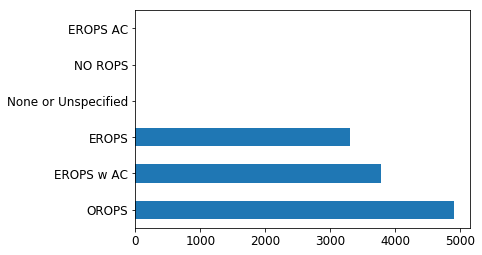In [ ]:
flds = ['Enclosure', 'SalePrice', 'pred', 'pred_std']
enc_summ = x[flds].groupby('Enclosure', as_index=False).mean()
enc_summ

Out[ ]:
Enclosure SalePrice pred pred_std
0 EROPS 9.849178 9.845237 0.276256
1 EROPS AC NaN NaN NaN
2 EROPS w AC 10.623971 10.579465 0.261992
3 NO ROPS NaN NaN NaN
4 None or Unspecified NaN NaN NaN
5 OROPS 9.682064 9.684717 0.220889
In [ ]:
enc_summ = enc_summ[~pd.isnull(enc_summ.SalePrice)]
enc_summ.plot('Enclosure', 'SalePrice', 'barh', xlim=(0,11));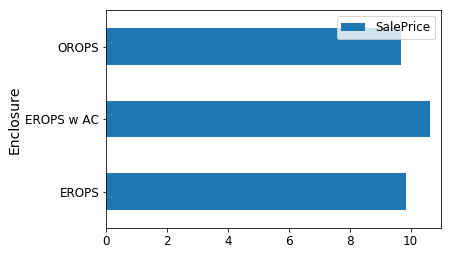In [ ]:
enc_summ.plot('Enclosure', 'pred', 'barh', xerr='pred_std', alpha=0.6, xlim=(0,11));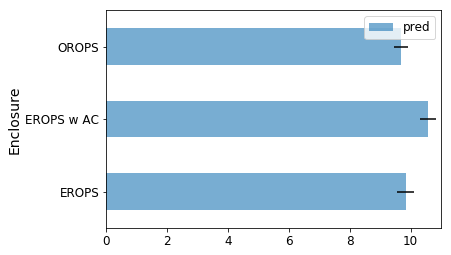Question: Why are the predictions nearly exactly right, but the error bars are quite wide?

In [ ]:
raw_valid.ProductSize.value_counts().plot.barh();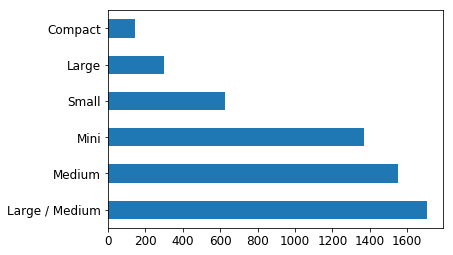In [ ]:
flds = ['ProductSize', 'SalePrice', 'pred', 'pred_std']
summ = x[flds].groupby(flds).mean()
summ

Out[ ]:
SalePrice pred pred_std
ProductSize
Compact 9.735093 9.888354 0.339142
Large 10.470589 10.392766 0.362407
Large / Medium 10.691871 10.639858 0.295774
Medium 10.681511 10.620441 0.285992
Mini 9.535147 9.555066 0.250787
Small 10.324448 10.322982 0.315314
In [ ]:
(summ.pred_std/summ.pred).sort_values(ascending=False)

Out[ ]:
ProductSize
Large             0.034871
Compact           0.034297
Small             0.030545
Large / Medium    0.027799
Medium            0.026928
Mini              0.026247
dtype: float64

# Feature importance¶

It's not normally enough to just to know that a model can make accurate predictions - we also want to know how it's making predictions. The most important way to see this is with feature importance.

In [ ]:
fi = rf_feat_importance(m, df_trn); fi[:10]

Out[ ]:
cols imp
37 Coupler_System 0.114632
13 ProductSize 0.103073
14 fiProductClassDesc 0.081206
2 ModelID 0.060495
39 Hydraulics_Flow 0.051222
63 saleElapsed 0.050837
10 fiSecondaryDesc 0.038329
19 Enclosure 0.034592
8 fiModelDesc 0.030848
In [ ]:
fi.plot('cols', 'imp', figsize=(10,6), legend=False);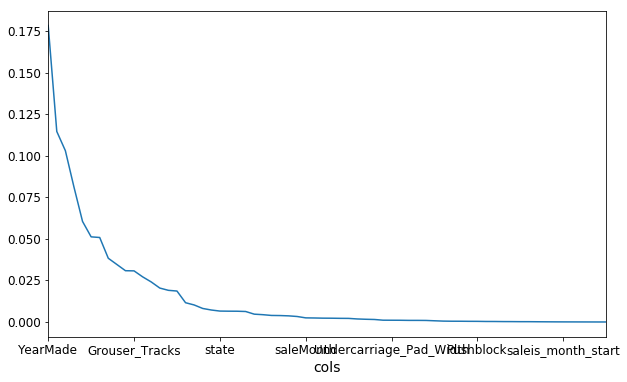In [ ]:
def plot_fi(fi): return fi.plot('cols', 'imp', 'barh', figsize=(12,7), legend=False)

In [ ]:
plot_fi(fi[:30]);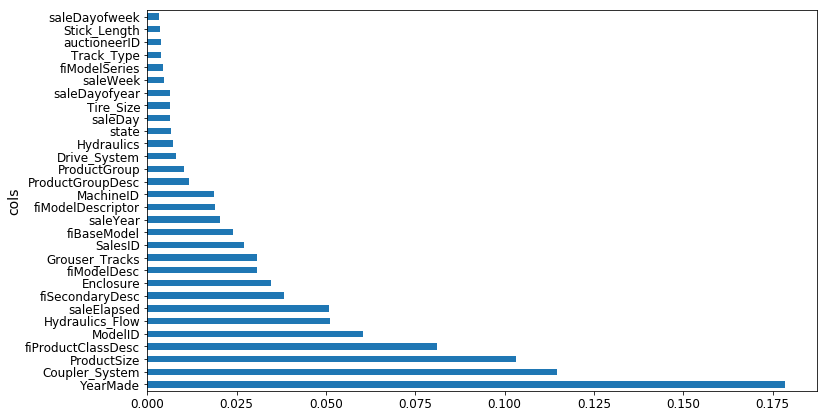In [ ]:
to_keep = fi[fi.imp>0.005].cols; len(to_keep)

Out[ ]:
24
In [ ]:
df_keep = df_trn[to_keep].copy()
X_train, X_valid = split_vals(df_keep, n_trn)

In [ ]:
m = RandomForestRegressor(n_estimators=40, min_samples_leaf=3, max_features=0.5,
n_jobs=-1, oob_score=True)
m.fit(X_train, y_train)
print_score(m)

[0.20685390156773095, 0.24454842802383558, 0.91015213846294174, 0.89319840835270514, 0.8942078920004991]

In [ ]:
fi = rf_feat_importance(m, df_keep)
plot_fi(fi);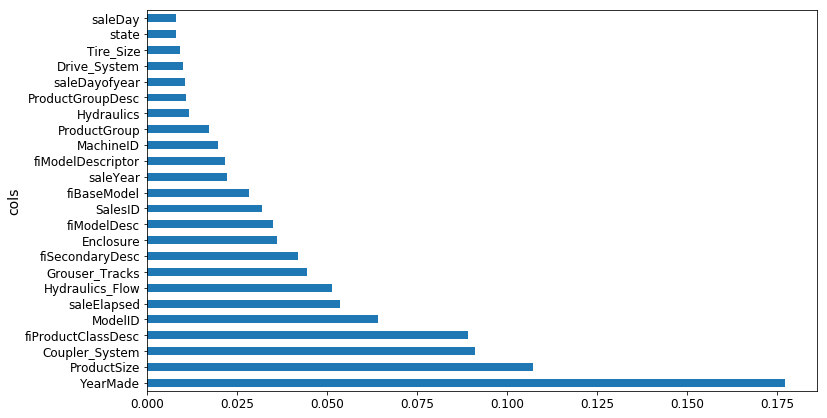## One-hot encoding¶

proc_df's optional max_n_cat argument will turn some categorical variables into new columns.

For example, the column ProductSize which has 6 categories:

• Large
• Large / Medium
• Medium
• Compact
• Small
• Mini

gets turned into 6 new columns:

• ProductSize_Large
• ProductSize_Large / Medium
• ProductSize_Medium
• ProductSize_Compact
• ProductSize_Small
• ProductSize_Mini

and the column ProductSize gets removed.

It will only happen to columns whose number of categories is no bigger than the value of the max_n_cat argument.

Now some of these new columns may prove to have more important features than in the earlier situation, where all categories were in one column.

In [ ]:
df_trn2, y_trn, nas = proc_df(df_raw, 'SalePrice', max_n_cat=7)
X_train, X_valid = split_vals(df_trn2, n_trn)

m = RandomForestRegressor(n_estimators=40, min_samples_leaf=3, max_features=0.6, n_jobs=-1, oob_score=True)
m.fit(X_train, y_train)
print_score(m)

[0.2132925755978791, 0.25212838463780185, 0.90966193351324276, 0.88647501408921581, 0.89194147155121262]

In [ ]:
fi = rf_feat_importance(m, df_trn2)
plot_fi(fi[:25]);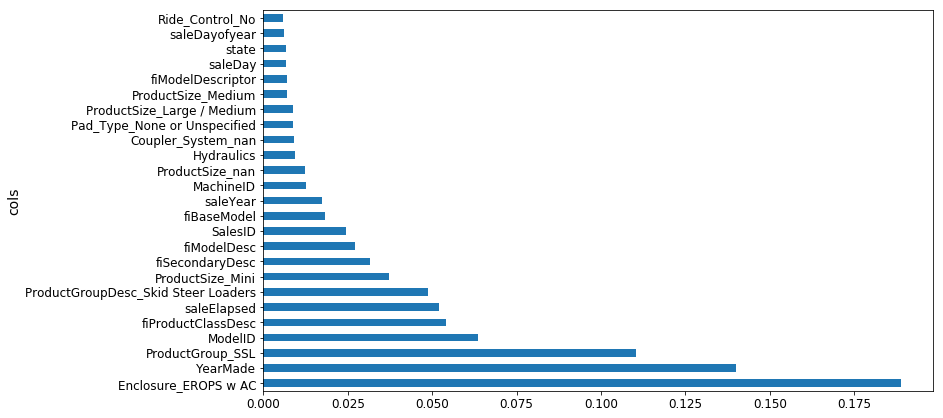# Removing redundant features¶

One thing that makes this harder to interpret is that there seem to be some variables with very similar meanings. Let's try to remove redundent features.

In [ ]:
from scipy.cluster import hierarchy as hc

In [ ]:
corr = np.round(scipy.stats.spearmanr(df_keep).correlation, 4)
corr_condensed = hc.distance.squareform(1-corr)
fig = plt.figure(figsize=(16,10))
dendrogram = hc.dendrogram(z, labels=df_keep.columns, orientation='left', leaf_font_size=16)
plt.show()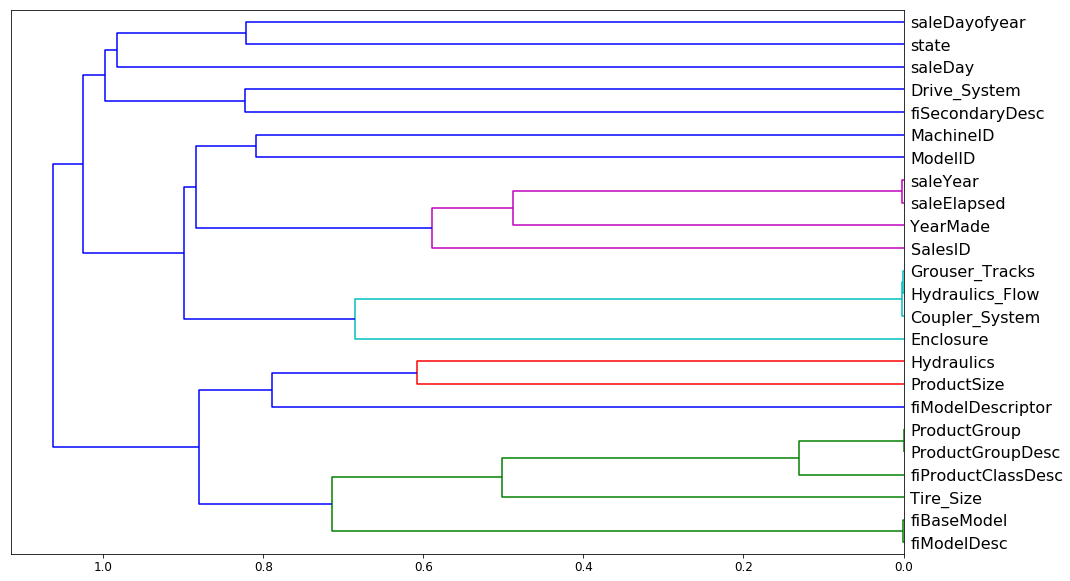Let's try removing some of these related features to see if the model can be simplified without impacting the accuracy.

In [ ]:
def get_oob(df):
m = RandomForestRegressor(n_estimators=30, min_samples_leaf=5, max_features=0.6, n_jobs=-1, oob_score=True)
x, _ = split_vals(df, n_trn)
m.fit(x, y_train)
return m.oob_score_


Here's our baseline.

In [ ]:
get_oob(df_keep)

Out[ ]:
0.88999425494301454

Now we try removing each variable one at a time.

In [ ]:
for c in ('saleYear', 'saleElapsed', 'fiModelDesc', 'fiBaseModel', 'Grouser_Tracks', 'Coupler_System'):
print(c, get_oob(df_keep.drop(c, axis=1)))

saleYear 0.889037446375
saleElapsed 0.886210803445
fiModelDesc 0.888540591321
fiBaseModel 0.88893958239
Grouser_Tracks 0.890385236272
Coupler_System 0.889601052658


It looks like we can try one from each group for removal. Let's see what that does.

In [ ]:
to_drop = ['saleYear', 'fiBaseModel', 'Grouser_Tracks']
get_oob(df_keep.drop(to_drop, axis=1))

Out[ ]:
0.88858458047200739

Looking good! Let's use this dataframe from here. We'll save the list of columns so we can reuse it later.

In [ ]:
df_keep.drop(to_drop, axis=1, inplace=True)
X_train, X_valid = split_vals(df_keep, n_trn)

In [ ]:
np.save('tmp/keep_cols.npy', np.array(df_keep.columns))

In [ ]:
keep_cols = np.load('tmp/keep_cols.npy')
df_keep = df_trn[keep_cols]


And let's see how this model looks on the full dataset.

In [ ]:
reset_rf_samples()

In [ ]:
m = RandomForestRegressor(n_estimators=40, min_samples_leaf=3, max_features=0.5, n_jobs=-1, oob_score=True)
m.fit(X_train, y_train)
print_score(m)

[0.12615142089579687, 0.22781819082173235, 0.96677727309424211, 0.90731173105384466, 0.9084359846323049]


# Partial dependence¶

In [ ]:
from pdpbox import pdp
from plotnine import *

In [ ]:
set_rf_samples(50000)


This next analysis will be a little easier if we use the 1-hot encoded categorical variables, so let's load them up again.

In [ ]:
df_trn2, y_trn, nas = proc_df(df_raw, 'SalePrice', max_n_cat=7)
X_train, X_valid = split_vals(df_trn2, n_trn)
m = RandomForestRegressor(n_estimators=40, min_samples_leaf=3, max_features=0.6, n_jobs=-1)
m.fit(X_train, y_train);

In [ ]:
plot_fi(rf_feat_importance(m, df_trn2)[:10]);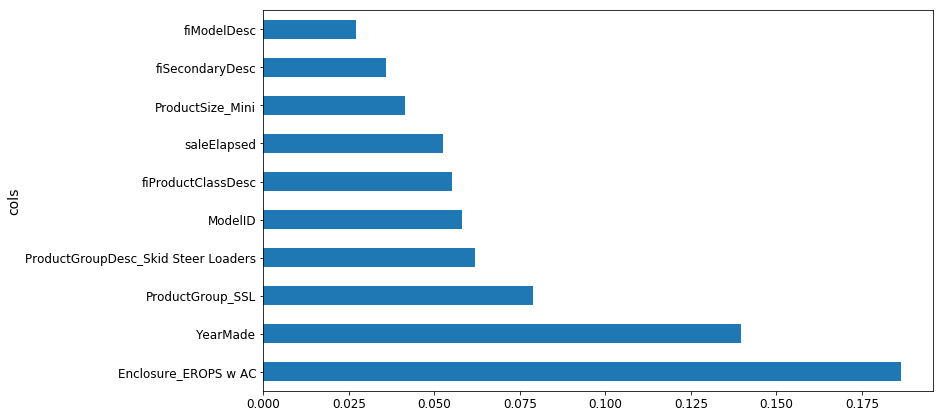In [ ]:
df_raw.plot('YearMade', 'saleElapsed', 'scatter', alpha=0.01, figsize=(10,8));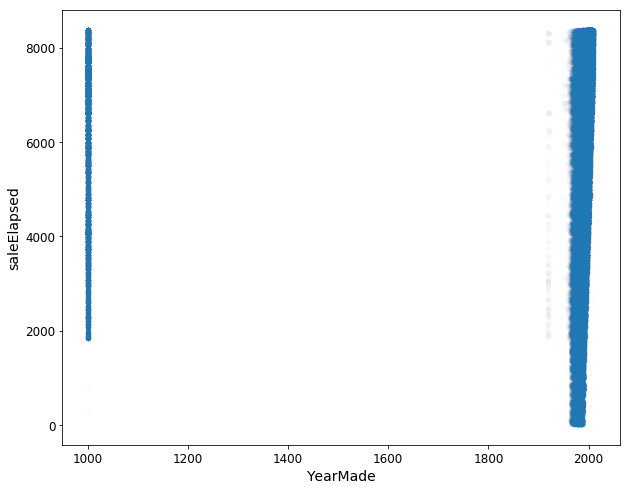In [ ]:
x_all = get_sample(df_raw[df_raw.YearMade>1930], 500)

In [ ]:
ggplot(x_all, aes('YearMade', 'SalePrice'))+stat_smooth(se=True, method='loess')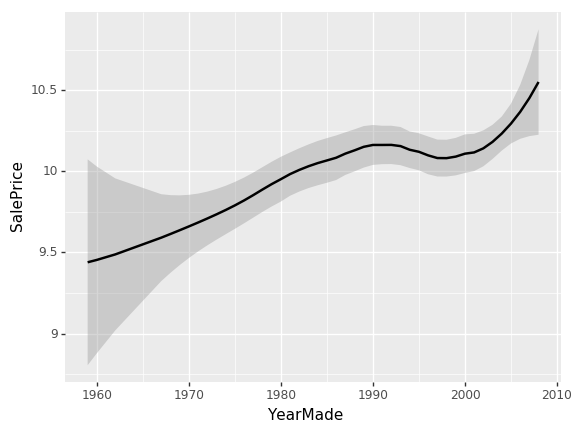Out[ ]:
<ggplot: (8729550331912)>
In [ ]:
x = get_sample(X_train[X_train.YearMade>1930], 500)

In [ ]:
def plot_pdp(feat, clusters=None, feat_name=None):
feat_name = feat_name or feat
p = pdp.pdp_isolate(m, x, feat)
return pdp.pdp_plot(p, feat_name, plot_lines=True,
cluster=clusters is not None,
n_cluster_centers=clusters)

In [ ]:
plot_pdp('YearMade')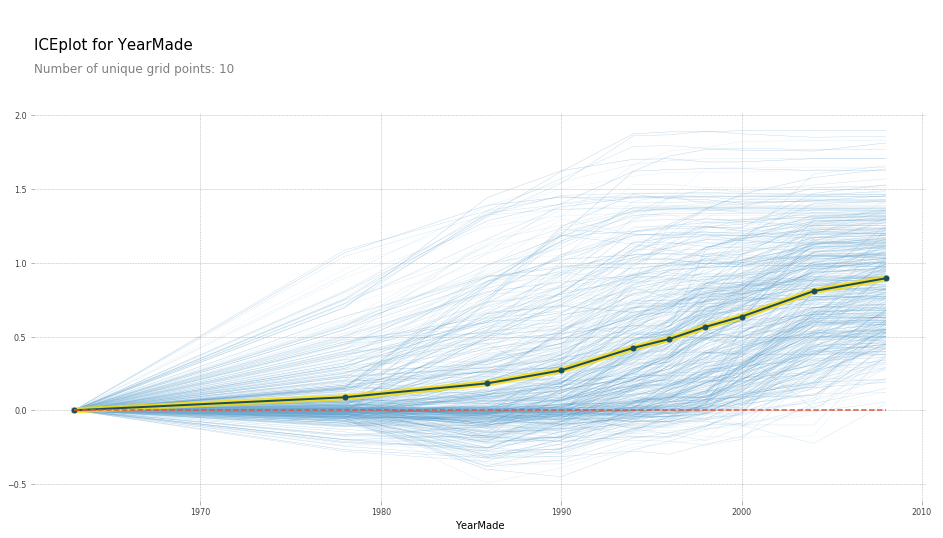In [ ]:
plot_pdp('YearMade', clusters=5)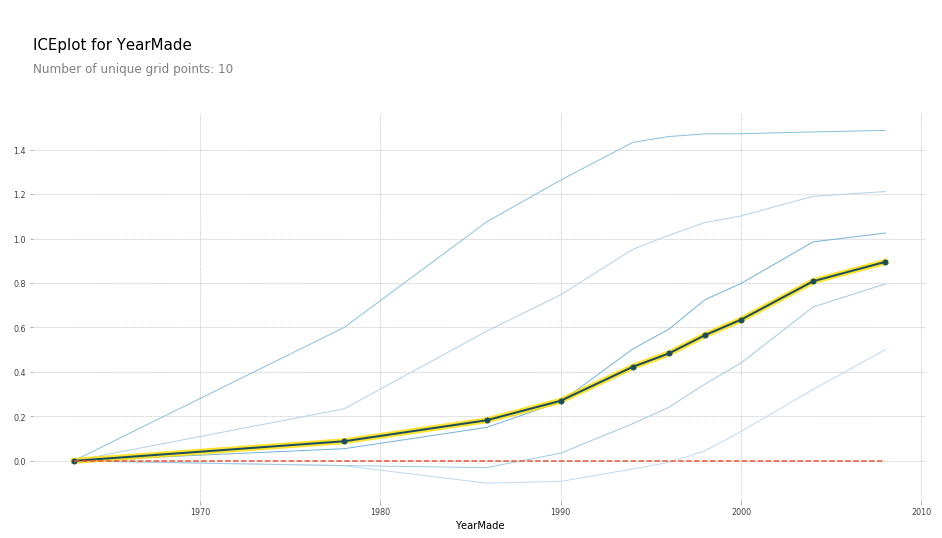In [ ]:
feats = ['saleElapsed', 'YearMade']
p = pdp.pdp_interact(m, x, feats)
pdp.pdp_interact_plot(p, feats)